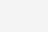# MySQL-优化示例

MySQL-优化示例

#### 1）引入案例

``````# 创建新表
create table test03
(
a1 int(4) not null,
a2 int(4) not null,
a3 int(4) not null,
a4 int(4) not null
);
# 创建一个复合索引
create index a1_a2_a3_test03 on test03(a1,a2,a3);
# 查看执行计划
explain select a3 from test03 where a1=1 and a2=2 and a3=3;
``````

``````# 查看执行计划
explain select a3 from test03 where a3=1 and a2=2 and a1=3;
``````

``````# 查看执行计划
explain select a3 from test03 where a1=1 and a3=2 group by a3;
``````

#### 2）单表优化

``````# 创建新表
create table book
(
bid int(4) primary key,
name varchar(20) not null,
authorid int(4) not null,
publicid int(4) not null,
typeid int(4) not null
);
# 插入数据
insert into book values(1,'tjava',1,1,2) ;
insert into book values(2,'tc',2,1,2) ;
insert into book values(3,'wx',3,2,1) ;
insert into book values(4,'math',4,2,3) ;
``````

``````explain
select bid from book
where typeid in(2,3) and authorid=1
order by typeid desc ;
``````

``````from .. on.. join ..where ..group by ..having ..select dinstinct ..order by ..limit ..
``````

① 优化1：基于此，我们进行索引的添加，并再次查看执行计划。

``````# 添加索引
create index typeid_authorid_bid on book(typeid,authorid,bid);
# 再次查看执行计划
explain
select bid from book
where typeid in(2,3) and authorid=1
order by typeid desc ;
``````

② 优化2：使用了in有时候会导致索引失效，基于此有了如下一种优化思路。

``````# 删除以前的索引
drop index typeid_authorid_bid on book;
# 再次创建索引
create index authorid_typeid_bid on book(authorid,typeid,bid);
# 再次查看执行计划
explain
select bid from book
where authorid=1  and typeid in(2,3)
order by typeid desc ;
``````

b.索引需要逐步优化(每次创建新索引，根据情况需要删除以前的废弃索引)

c.将含In的范围查询，放到where条件的最后，防止失效。

``````explain select bid from book
where  authorid=1 and typeid =3
order by typeid desc ;
``````#### 3）两表优化

``````# 创建teacher2新表
create table teacher2
(
tid int(4) primary key,
cid int(4) not null
);
# 插入数据
insert into teacher2 values(1,2);
insert into teacher2 values(2,1);
insert into teacher2 values(3,3);
# 创建course2新表
create table course2
(
cid int(4) ,
cname varchar(20)
);
# 插入数据
insert into course2 values(1,'java');
insert into course2 values(2,'python');
insert into course2 values(3,'kotlin');
``````

``````explain
select *
from teacher2 t
left outer join course2 c
on t.cid=c.cid
where c.cname='java';
``````① 优化对于两张表，索引往哪里加？答：对于表连接，小表驱动大表。索引建立在经常使用的字段上。为什么小表驱动大表好一些呢？

``````    小表:10
大表:300
# 小表驱动大表
select ...where 小表.x10=大表.x300 ;
for(int i=0;i<小表.length10;i++)
{
for(int j=0;j<大表.length300;j++)
{
...
}
}
# 大表驱动小表
select ...where 大表.x300=小表.x10 ;
for(int i=0;i<大表.length300;i++)
{
for(int j=0;j<小表.length10;j++)
{
...
}
}
``````

``````# 给左表的字段加索引
create index cid_teacher2 on teacher2(cid);
# 查看执行计划
explain
select *
from teacher2 t
left outer join course2 c
on t.cid=c.cid
where c.cname='java';
````````````# 给cname的字段加索引
create index cname_course2 on course2(cname);
# 查看执行计划
explain
select t.cid,c.cname
from teacher2 t
left outer join course2 c
on t.cid=c.cid
where c.cname='java';
``````(0)

4550

4880

4360

4670

4330

4271